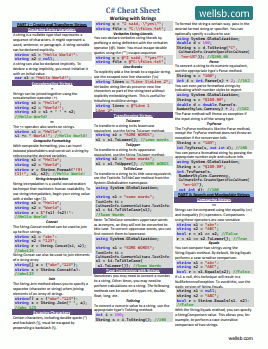# C# Foreach Loop Through Array

## HOW TO LOOP THROUGH AN ARRAY IN C#

by

An important advantage of arrays in C# is the ability to use a `for` loop or `foreach` loop to cycle through the elements of the array.

Suppose you have declared an array of integers and you want to know which variable contains a specific value. Because your data is structured in an array, you can loop through the array's elements in order to find the value you are interested in.

## For Loop Through Array

There are two approaches for looping through the elements of an array. The first approach will use a `for` loop, which we have learned about in a previous lesson.

``````string[] firstNames = new string[] { "Brad", "Brian", "Bob", "Bill" };

for (int i = 0; i < firstNames.Length; i++)
{
Console.WriteLine(firstNames[i]);
}
``````

In this example, we want to write the values of four string variables to the console. We have declared the strings in a string[] array. We know the array contains four elements: firstNames, firstNames, firstNames, and firstNames.

Remember, the `for` loop syntax, shown in Line 3, has three parts - the initializer, condition, and iterator. First, we will initialize a counter variable that will represent the index values of our array elements. Indexing begins with a base of 0, so we will set the initial value `i = 0`.

Next, we want the program to loop until we reach the end of the array. In this case, it is easy to see that our array has four elements. However, we may not always know how many elements are in the array we are working with. Fortunately, C# arrays have a Length property we can use to get the array's length. We access the property using `arrayName.Length`, where arrayName is the name of our array. In this case, our array is called firstNames and contains four elements, so firstNames.Length returns a value of 4. Our loop will continue as long as `i < 4`.

Finally, we increment the counter variable by one using the `i++` increment operator.

Within the `for` loop, we use Console.WriteLine() to print the value of the element in the array that is currently being accessed. When i = 0, our program will print the value corresponding to firstNames, which is "Brad." As `i` is incremented, our program will print the successive values in the array.

The `for` loop is useful when you know (or need to know) the indices of the elements you want to manipulate. For example, you may use this loop if you want to manipulate every nth element of an array or if you want to know that "Bob" is the third element in the array.

## Foreach Loop Through Array

The second type of loop for working with arrays is the `foreach` loop.

``````string[] firstNames = new string[] { "Brad", "Brian", "Bob", "Bill" };

foreach (string firstName in firstNames)
{
Console.WriteLine(firstName);
}
``````

Here, we are using a `foreach` loop to iterate through the elements of our array. On Line 3, we see the `foreach` syntax.

``````foreach (dataType tempVar in arrayName){}
``````

I have replaced the first keyword, dataType, with the type of variable I am storing in my array. In this case, they are string variables.

The second keyword, tempVar, is arbitrary. You can call it anything you want. I have elected to use the naming convention where I use the singular version of my array name as the name of this temporary variable. The `foreach` loop will iterate through each item in the array, and temporarily copy the current element to the tempVar variable.

The final keyword, arrayName, is the name of the array we are interested in looping through. In this case, our array is called firstNames.

Inside the `foreach` loop, we will again print the values of all the elements in our array to the screen. This time, because the current element is copied to the temporary variable, we can simply reference the elements using firstName to do whatever we want.

## The Bottom Line

In this tutorial, you learned two methods for iterating through the elements of an array. The `for` loop is most helpful when you know or need to know the index positions of the items you want to work with. In most other cases, the `foreach` loop is a less complicated option.

Can you think of any cool uses for looping through arrays? Let me know in the comments!

#### Don't stop learning!